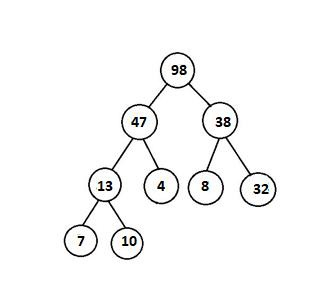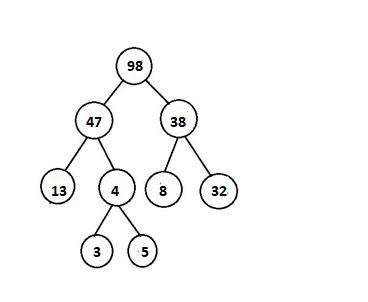# Check if a given Binary Tree is Heap in C++

## Concept

With respect of a given binary tree, we need to verify whether it has heap property or not, Binary tree need to satisfy the following two conditions for being a heap –

• Binary tree should be a complete tree (i.e. all levels except last should be full).

• Binary tree's every node’s value should be greater than or equal to its child node (considering max-heap).

Example

With respect of following example this tree contains heap property –The following example does not have heap property –## Method

It is required to verify each of the above condition separately, for verifying completeness isComplete(This function checks if the binary tree is complete or not) and for verifying heap isHeapUtil function are written.

With respect of writing isHeapUtil function, we consider the following things –

• Each and every Node can have 2 children, 0 child (last level nodes) or 1 child (there can be maximum one such node).

• If it has been seen that Node has No child then it’s a leaf node and returns true(Base case)

• If it has been seen that Node has one child (it must be left child because it is a complete tree) then we require to compare this node with its single child only.

• If it has been seen that the Node has both child then verify heap property at Node at recur for both subtrees.

## Example

Live Demo

/* C++ program to checks if a binary tree is max heap or not */
#include <bits/stdc++.h>
using namespace std;
struct Node1{
int key;
struct Node1 *left;
struct Node1 *right;
};
struct Node1 *newNode(int k){
struct Node1 *node1 = new Node1;
node1->key = k;
node1->right = node1->left = NULL;
return node1;
}
unsigned int countNodes(struct Node1* root1){
if (root1 == NULL)
return (0);
return (1 + countNodes(root1->left) + countNodes(root1->right));
}
bool isCompleteUtil (struct Node1* root1, unsigned int index1, unsigned int number_nodes){
if (root1 == NULL)
return (true);
if (index1 >= number_nodes)
return (false);
// Recur for left and right subtrees
return (isCompleteUtil(root1->left, 2*index1 + 1, number_nodes) && isCompleteUtil(root1->right, 2*index1 + 2, number_nodes));
}
bool isHeapUtil(struct Node1* root1){
if (root1->left == NULL && root1->right == NULL)
return (true);
if (root1->right == NULL){
return (root1->key >= root1->left->key);
}
else{
if (root1->key >= root1->left->key &&
root1->key >= root1->right->key)
return ((isHeapUtil(root1->left)) &&
(isHeapUtil(root1->right)));
else
return (false);
}
}
bool isHeap(struct Node1* root1){
unsigned int node_count = countNodes(root1);
unsigned int index1 = 0;
if (isCompleteUtil(root1, index1, node_count) &&
isHeapUtil(root1))
return true;
return false;
}
// Driver program
int main(){
struct Node1* root1 = NULL;
root1 = newNode(10);
root1->left = newNode(9);
root1->right = newNode(8);
root1->left->left = newNode(7);
root1->left->right = newNode(6);
root1->right->left = newNode(5);
root1->right->right = newNode(4);
root1->left->left->left = newNode(3);
root1->left->left->right = newNode(2);
root1->left->right->left = newNode(1);
if (isHeap(root1))
cout << "Given binary tree is a Heap\n";
else
cout << "Given binary tree is not a Heap\n";
return 0;
}

## Output

Given binary tree is a Heap